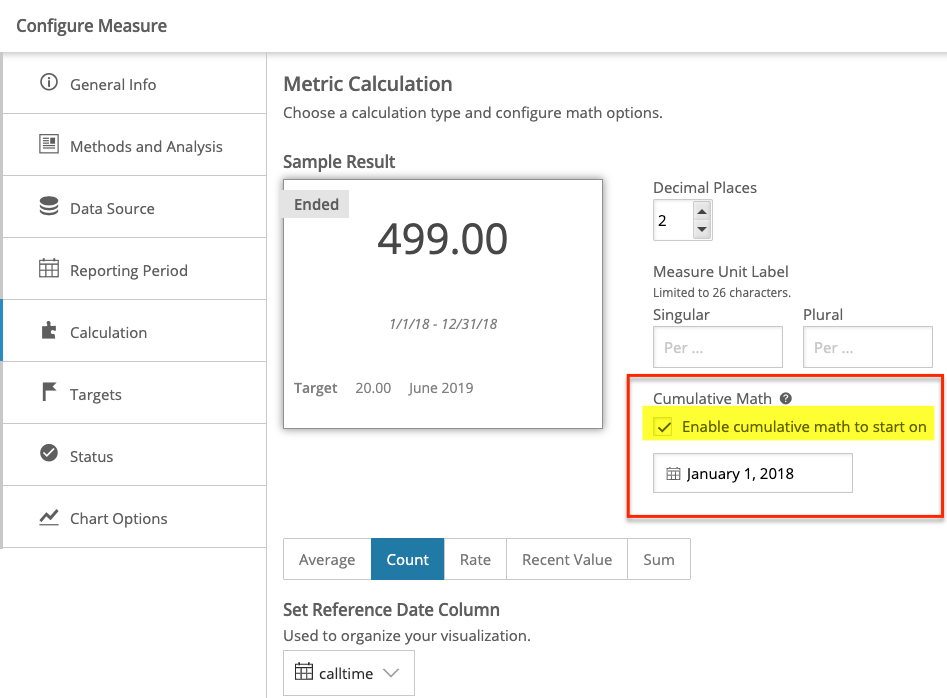# Define a Measure Calculation

This article covers the options in the Calculation tab of a Measure configuration. For instructions on how to configure the rest of your Measure, check out this article!

You can choose among average, count, rate, recent value, sum, and cumulative math to calculate your Measure. All calculation types support in-line filtering, so you are not required to create a filtered view of a dataset before building your Measure.

Note: No matter the calculation type chosen, you must select a date/time column as your reference date.

## Average

The average calculation type sums the rows for the numeric column you select, and divides this sum by the count of rows.

## Count

This calculation type counts the number of rows within a column of the your dataset. This calculation type will count numeric and categorical data. Note that checking the "Include missing or null values" will skip over (not count) cells that do not contain a value.

## Rate

Rate calculates a rate or percentage by dividing the value of a numerator by the value of a denominator. The selected numerator or denominator columns can be summed or counted, and you also have the option to add filters to either or both of these columns (for examples of how to use filtering in your numerator or denominator, see our release notes). You can also enter an exact value as the denominator rather than selecting a column from your dataset, and to ignore null values.

When calculating a rate, if you choose a column that results in your denominator being zero, you will see a notification in the sample fraction displayed in a gray box on the right. See this article for more about error messaging.

## Recent Value

Recent value displays the most recent value in a column, given the condition and date column selected.

## Sum

Sum displays the sum of a selected column in your dataset. This calculation type can only be used on numeric columns.

## Cumulative Math

Cumulative math allows you to sum something across multiple reporting periods. This is very useful for performance programs that rely on a running count of something to determine program outcomes. Cumulative math is available across all calculation types, except for Recent Value.

To enable cumulative math, select the "Cumulative Math" checkbox on the right-hand side of the screen within the Calculation tab.For an example of how to use cumulative math, see the release notes here.